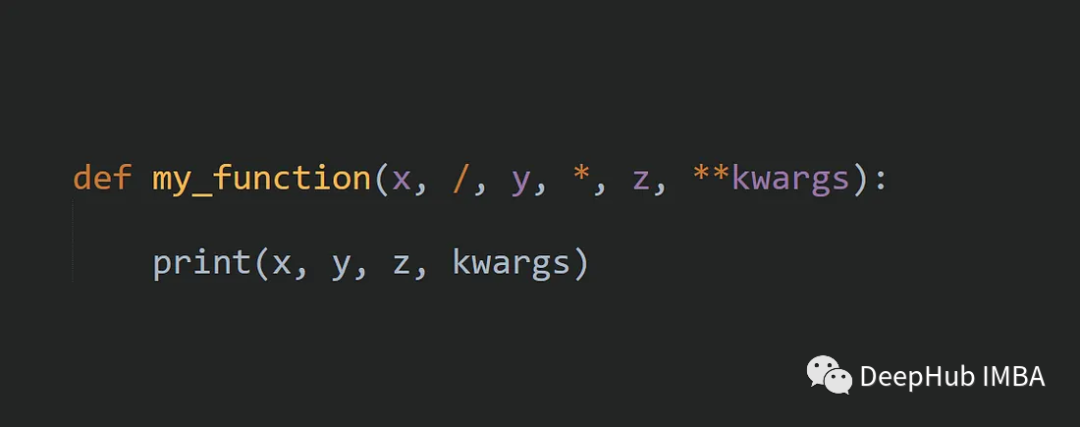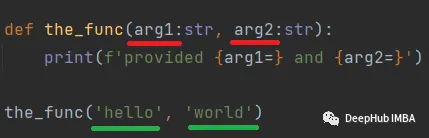0

# Python中函数参数传递方法*args, **kwargs，还有其他## 定义和传递参数

parameters 和arguments 之间的区别是什么?

• Parameters 是函数定义中定义的名称
• Arguments是传递给函数的值`````` defthe_func(greeting, thing):
print(greeting+' '+thing)

the_func('hello', thing='world')
``````

`````` the_func('hello', 'world')                  # -> 'hello world'
the_func('world', 'hello')                  # -> 'world hello'
the_func(greeting='hello', thing='world')   # -> 'hello world'
the_func(thing='world', greeting='hello')   # -> 'hello world'
the_func('hello', thing='world')            # -> 'hello world'
``````

## 函数参数

1、如何获得所有未捕获的位置参数

`````` defmultiply(a, b, *args):
result=a*b
forarginargs:
result=result*arg
returnresult
``````

`````` multiply(1, 2)          # returns 2
multiply(1, 2, 3, 4)    # returns 24
``````

2、如何获得所有未捕获的关键字参数

`````` defintroduce(firstname, lastname, **kwargs):
introduction=f"I am {firstname}{lastname}"
forkey, valueinkwargs.items():
introduction+=f" my {key} is {value} "
returnintroduction
``````

**kwargs关键字会将所有不匹配的关键字参数存储在一个名为kwargs的字典中。然后可以像上面的函数一样访问这个字典。

`````` print(introduce(firstname='mike', lastname='huls'))
# returns "I am mike huls"

print(introduce(firstname='mike', lastname='huls', age=33, website='mikehuls.com'))
# I am mike huls my age is 33  my website is overfit.cn
``````

3、如果想只接受关键字参数，那怎么设计

`````` deftransfer_money(*, from_account:str, to_account:str, amount:int):
print(f'Transfering \${amount} FORM {from_account} to {to_account}')

transfer_money(from_account='1234', to_account='6578', amount=9999)
# won't work: TypeError: transfer_money() takes 0 positional arguments but 1 positional argument (and 2 keyword-only arguments) were given
transfer_money('1234', to_account='6578', amount=9999)
# won't work: TypeError: transfer_money() takes 0 positional arguments but 3 were given
transfer_money('1234', '6578', 9999)
``````

4、如何设计函数只接受位置参数

`````` defthe_func(arg1:str, arg2:str, /):
print(f'provided {arg1=}, {arg2=}')

# These work:
the_func('num1', 'num2')
the_func('num2', 'num1')

# won't work: TypeError: the_func() got some positional-only arguments passed as keyword arguments: 'arg1, arg2'
the_func(arg1='num1', arg2='num2')
# won't work: TypeError: the_func() got some positional-only arguments passed as keyword arguments: 'arg2'
the_func('num1', arg2='num2')
``````

`````` defexceeds_100_bytes(x, /) ->bool:
returnx.__sizeof__() >100

exceeds_100_bytes('a')
exceeds_100_bytes({'a'})
``````

``````def len(__obj: Sized) -> int:
``````

5、混合和匹配

`````` deflen_new(x, /, *, no_duplicates=False):
if (no_duplicates):
returnlen(list(set([aforainx])))
returnlen(x)
``````

`````` print(len_new('aabbcc'))                                  # returns 6
print(len_new('aabbcc', no_duplicates=True))              # returns 3
print(len_new([1, 1, 2, 2, 3, 3], no_duplicates=False))   # returns 6
print(len_new([1, 1, 2, 2, 3, 3], no_duplicates=True))    # returns 3

# Won't work: TypeError: len_() got some positional-only arguments passed as keyword arguments: 'x'
print(len_new(x=[1, 1, 2, 2, 3, 3]))
# Won't work: TypeError: len_new() takes 1 positional argument but 2 were given
print(len_new([1, 1, 2, 2, 3, 3], True))
``````

6、最后把它们合在一起

`````` defthe_func(pos_only1, pos_only2, /, pos_or_kw1, pos_or_kw2, *, kw1, kw2, **extra_kw):
# cannot be passed kwarg   <--   | --> can be passed 2 ways | --> can only be passed by kwarg
print(f"{pos_only1=}, {pos_only2=}, {pos_or_kw1=}, {pos_or_kw2=}, {kw1=}, {kw2=}, {extra_kw=}")
``````

`````` # works (pos_or_kw1 & pow_or_k2 can be passed positionally and by kwarg)
pos_only1='pos1', pos_only2='pos2', pos_or_kw1='pk1', pos_or_kw2='pk2', kw1='kw1', kw2='kw2', extra_kw={}
pos_only1='pos1', pos_only2='pos2', pos_or_kw1='pk1', pos_or_kw2='pk2', kw1='kw1', kw2='kw2', extra_kw={}
pos_only1='pos1', pos_only2='pos2', pos_or_kw1='pk1', pos_or_kw2='pk2', kw1='kw1', kw2='kw2', extra_kw={'kw_extra1': 'extra_kw1'}

# doesnt work, (pos1 and pos2 cannot be passed with kwarg)
# the_func(pos_only1='pos1', pos_only2='pos2', pos_or_kw1='pk1', pos_or_kw2='pk2', kw1='kw1', kw2='kw2')

# doesnt work, (kw1 and kw2 cannot be passed positionally)
# the_func('pos1', 'pos2', 'pk1', 'pk2', 'kw1', 'kw2')
``````

## 总结

`````` deffunc(x,/,y,*,z,**k):
``````
``````(x,/,y,*,z,**k)
``````

:是函数的参数。总共有四个参数:

• `x`: 是一个常规参数，这意味着它可以按位置传递，也可以按关键字传递。
• `/,`: 是一个参数分隔符，将仅限位置的参数与其他参数分开。与前面的`x`结合，意味着`x`只能按位置传递。
• `y`: 时另一个常规参数。
• `*`: 是一个参数分隔符，用于分隔仅限位置参数和仅限关键字参数。它意味着后面的`z`只能通过关键字传递。
• `z`: 是一个仅限关键字的参数。
• `**k`: 这是一个参数，将所有剩余的关键字参数收集到一个名为' k '的字典中。

1、尽量不要在函数定义中将可变位置参数 *args 和可变关键字参数 **kwargs 放在一起，因为这样会让函数的调用方式变得不太直观。

2、在使用可变参数时，要保证函数的行为是可预测的。上面函数中的进行了太多的python语法糖，对于理解该函数的参数会造成很大的困惑，也就是可读性太差，我们在进行codereview（如果你了解什么是codereview就说，不了解就说组长检查）/组长merge代码 时会直接要求返工，所以我们在实际开发时是不会用这个的。

### “Python中函数参数传递方法*args, **kwargs，还有其他”的评论:

##### 关于作者##### Deephub